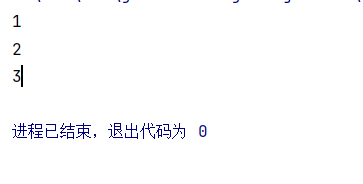# Java方法

1.什么是方法

2.方法上定义及调用

3.方法重载

4.可变参数

### 1.什么是方法

System.out.println();是什么？
System是系统的一个类
out是一个输出对象
println()就是一个方法

• 方法是语句的集合，他们在一起执行一个功能
• 方法是解决一类问题的步骤的有序组合
• 方法包含于类或对象中
• 方法在程序中被创建，在其他地方被引用
• 方法设计的原则：方法的本意是功能块，就是实现某个功能的语句块的集合。设计方法的时候最好保持方法的原子性：一个方法只能完成一个功能。

public class Demo01 {
//main方法
//public static ：修饰符 ； void：返回值类型
public static void main(String[] args) {
System.out.println(sum);
}
//加法
public static int add(int a,int b){
return a + b;
}
}


运行结果### 2.方法的定义及调用

#### （1）.方法的定义

修饰符  返回值类型  方法名（参数类型  参数名）{
……
方法体
……
return 返回值；
}


Java的方法类似其他语言的函数，是一段用来完成特定功能的代码片段，一般情况下，定义一个方法包含以下语法

• 方法包含一个方法头和一个方法体，下面是一个方法的所有部分

• 修饰符：这是可选的，告诉编译器如何调用该方法，定义了该方法的访问类型

• 返回值类型：方法可能会有返回值。returnValue Type是方法返回值的数据类型。有些方法执行所需的操作，但没有返回值，这种情况下returnValue Type是关键字void。

• 方法名：是方法的实际名称。方法名和参数表共同构成方法签名

• 参数类型：参数像是一个占位符。当方法被调用时，传递值给参数。这个值被称为实参或变量。参数列表是指方法的参数类型、顺序和参数的个数。参数是可选的，方法可以不包括任何参数。

• 形式参数：在方法被调用时，用于接收外界输入的数据
• 实参：调用方法时，实际传递给方法的数据
• 方法体：方法体包含具体的语句，定义该方法的功能

public class Demo01 {

public static void main(String[] args) {
int sum = add(1,2); // 这里的1和2是实参
System.out.println(sum);
}
//加法
public static int add(int a,int b){//这里的a和b是形参
return a + b;
}
}


#### （2）.方法的调用

• 调用方法：对象名.方法名（实参列表）

• Java支持两种调用方法的方式，根据方法是否返回值来选择

• 当方法返回一个值的时候，方法调用通常被当做一个值

例：

 int larger  = max(10,20);

• 如果方法返回值是void，方法调用一定是一条语句

例：

System.out.println("Hello World !");


例:

import java.util.Scanner;

public class Demo02 {
public static void main(String[] args) {
Scanner scanner = new Scanner(System.in);
int i = scanner.nextInt();
int j = scanner.nextInt();
int max = max(i,j); //调用方法
System.out.println(max);
}

//比大小
public static int max(int num1,int num2){
int result = -1;

if (num1 == num2){
System.out.println("num1 == num2");
return 0; //终止方法
}

if (num1 > num2){
result = num1;
}else {
result = num2;
}

return result;
}
}


### 3.方法重载

• 重载就是在一个类中，有相同的函数名称，但形参不同的函数

• 方法的重载规则：

• 方法名称必须相同
• 参数列表必须不同（个数不同、类型不同或参数排列顺序不同等）
• 方法的返回类型可以相同可以不同
• 仅仅返回类型不同不足以成为方法的重载
• 实现理论

• 方法名称相同时，编译器会根据调用方法的参数个数、参数类型等去逐个匹配，以选择对应的方法，如果匹配失败，则编译器报错

例：

import java.util.Scanner;

public class Demo03 {
public static void main(String[] args) {
double max = max(10,20);
System.out.println(max);
}

//比大小（int型）
public static int max(int num1,int num2){
int result = -1;

if (num1 == num2){
System.out.println("num1 == num2");
return 0; //终止方法
}

if (num1 > num2){
result = num1;
}else {
result = num2;
}
return result;
}
//方法重载
//比大小（double型）
public static double max(double num1,double num2){
double result = -1;

if (num1 == num2){
System.out.println("num1 == num2");
return 0; //终止方法
}

if (num1 > num2){
result = num1;
}else {
result = num2;
}
return result;
}
}


运行结果例：方法重载，参数个数不同

    public static double max(double num1,double num2,double num3){
double result = -1;
if (num1 == num2){
System.out.println("num1 == num2");
return 0; //终止方法
}
if (num1 > num2){
result = num1;
}else {
result = num2;
}
return result;
}


### 4.可变参数

• JDK 1.5 开始，Java支持传递类型的可变参数给一个方法
• 在声明中，在指定参数类型后面加一个省略号（…）
• 一个方法只能指定一个可变参数，它必须是方法的最后一个参数。任何普通的参数必须在它之前声明

public class Demo04 {
public static void main(String[] args) {
Demo04 demo04 = new Demo04();
demo04.test(1,2,3);
}

public void test(int... i ){ //不定项参数
System.out.println(i);
System.out.println(i);
System.out.println(i);
}
}public class Demo04 {
public static void main(String[] args) {
//调用可变参数的方法
printMax(34,3,3,2,56,5);
printMax(new double[]{1,2,3});
}

public static void printMax(double...numbers){
if (numbers.length == 0){
System.out.println("No argument passed");
return;
}
double result = numbers;
for (int i = 1;i < numbers.length;i++){
if (numbers[i] > result){
result = numbers[i];
}
}
System.out.println("The max value is " + result);
}
}posted @ 2021-07-20 15:26  小芦荟同学  阅读(18)  评论(0编辑  收藏  举报